# FCFF vs FCFE

Free Cash Flow to the Firm (FCFF) and Free Cash Flow to Equity (FCFE) are two types of free cash flow. They are sometimes also referred to as the unlevered free cash flow and levered free cash flow, respectively. Both measures are used to perform free cash flow valuation. Unlike dividends, FCFE and FCFF reflect the firms capacity to pay dividends. The difference between the two cash flow measures is the type of cash flow used.

On this page we compare free cash flow to firm versus free cash flow to equity and implement both formulas using an Excel spreadsheet.

## Free Cash Flow to the Firm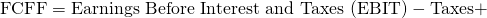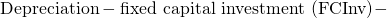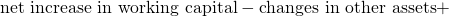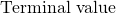The FCFF is the cash flow generated by a company before debt principal repayment but after reinvestment and taxes. It estimates the value of the company by discounting the FCFF by the weighted average cost of capital. It is the cash flow available to suppliers of capital. Note that this is not just the equity holders, but also the debt holders. FCFF is het cash available after paying all operating expenses, including taxes.

## Free Cash Flow to Equity

The Free Cash Flow to Equity (FCFE) is the cash flow after debt cash flows, capital investments, and taxes. This means that we now focus on the cash flow available to the equity holders. The FCFE formula equals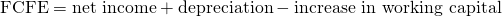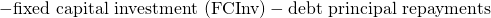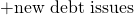It can also be expressed using the cash flow from operations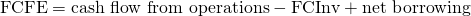## FCFF vs FCFE

There are two important differences between the FCFF and FCFE. While the FCFF reports the cash flow available to all providers of capital, the FCFE is narrower since it reports the cash flow available to equity holders only. Another difference is the discount rate used. When we using the FCFF or FCFE to estimate a firm’s value, we discount both measures. In the case of the FCFF, we use the weighted average cost of capital. In the case of FCFE, we use the cost of equity. Comparing FCFF vs FCFE, we notice that there are number of differences, especially when using them as inputs for a cash flow valuation model.

## FCFF to FCFE

It is also possible to calculate the Free Cash Flow to the Firm using the Free Cash Flow to Equity. The following formula can be used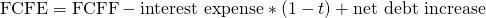where t is the tax rate.

FCFE and FCFE company valuation

To value a firm or the equity of the firm using the above two measures, we use the following set of formulas. First, we report the free cash flow valuation model using FCFF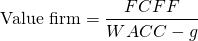where g is the growth rate of operating income

In the case of FCFE, we get the following free cash flow valuation model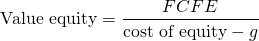where g is the growth rate in equity income (dividends).

### Free cash flow valuation model Excel

Want to have an implementation in Excel? Download the Excel file: FCFF vs FCFE